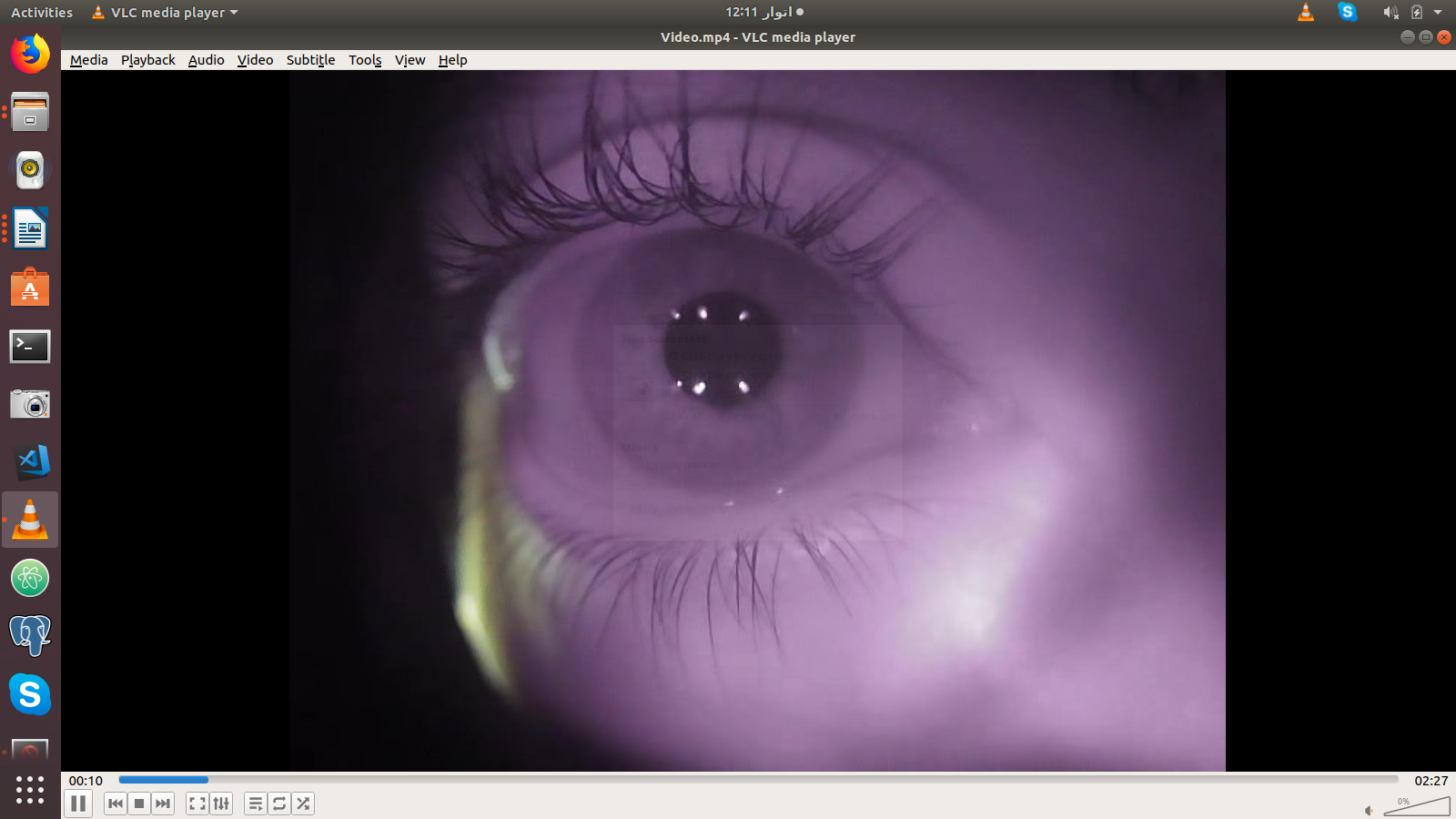## Video Processing in Python using OpenCV

##### In this part of Learning Python we Cover Video Processing In Python
Written by |30-Jul-2019 | 0 Comments | 681 Views

Now we will see a project in which we are going to demonstrate how we can recognize the cornea of an eye from the video.

The video has an image like this:Figure 1

And our task is to recognize the eye extract the pupil out of it, calculate the pupil diameter and also the central position of the pupil along X and Y axis. For eye recognition, we are going to use Haar cascade.

Haarcascade is an image classifier by which we can also train our classifier. But it is now an old technique it is not as good as Convolutional Neural Network CNN nowadays. It is one of the first types of classification technique develop in early in 2000. Many kinds of Haar cascades are available online like frontal face, eyes, cars, etc. So, let’s start the projects

At first, to make our code read the video streaming for that purpose following code is to be implemented:

`import numpy as np`
`import cv2`
`import matplotlib.pyplot as plt`
`import matplotlib.animation as animation`
`eye_cascade = cv2.CascadeClassifier('haarcascae_eye.xml')`
`cap = cv2.VideoCapture('Video.mp4')`

SourceCode Credit: Hassan Rehman, Pupil-Detection, (2018), GitHub repository, https://github.com/HassanRehman11/Pupil-Detection/blob/master/PupilDetection.py

First of all, we have imported NumPy, cv2, Matplotlib these modules we will be using in our project. After that, we initiate eye cascade. This cascade classifier will allow us to identify the eye in a video. Whenever the open eye is on the video, it will identify it. After that, we initiate the video that we have described it above

After that we will initialize some variable as follows:

`diameter=[]`
`blink =False`
`bcount = -1`
`kernel = np.ones((5,5),np.uint8)`
`global a`
`font = cv2.FONT_HERSHEY_SIMPLEX`

SourceCode Credit: Hassan Rehman, Pupil-Detection, (2018), GitHub repository, https://github.com/HassanRehman11/Pupil-Detection/blob/master/PupilDetection.py

We have begun the list of diameters so we can have all the data of diameter at the end to display it on Matplotlib. We initiate blink “False” whenever the blink “true” it will be seen on our screen. Then we have initialized the blink count it will be incremented after every blink. Kernel for some filtration technique and font for overlaying the font on the main screen.

`try:`
`    while 1:`
`        ret, img = cap.read()`
`        gray = cv2.cvtColor(img, cv2.COLOR_BGR2GRAY)`
`        eyes = eye_cascade.detectMultiScale(gray,1.1,7)`

SourceCode Credit: Hassan Rehman, Pupil-Detection, (2018), GitHub repository, https://github.com/HassanRehman11/Pupil-Detection/blob/master/PupilDetection.py

Now we have open try block and initialize the loop. We start reading the cap and convert the image into grayscale. That grayscale image is subject to eye cascade object which will then identify the eye.

` if (len(eyes)>0):`
`            a = "Eye Open"`
`            `
`            if (blink==True):`
`                blink=False`
`               `
`            cv2.putText(img,a,(10,30), font, 1,(0,0,255),2,cv2.LINE_AA)`

SourceCode Credit: Hassan Rehman, Pupil-Detection, (2018), GitHub repository, https://github.com/HassanRehman11/Pupil-Detection/blob/master/PupilDetection.py

Now the main thing starts. Whenever there is an eye mean there is a more than “0” eye in a frame and eye is open blink flag will be equal to “true” and text will be an overlay on the main screen. Put text will take the following arguments. The object of an image on which you want to display the text. The position on x and y-axis where you want to place the text the type of font which we have already initialized above. Then the color, here it will take a tuple of BGR, so we are initializing red color here and in last line flag.

`for (ex,ey,ew,eh) in eyes:`
`                #cv2.rectangle(img,(ex,ey),(ex+ew,ey+eh),(0,255,0),2)`
`                roi_gray2 = gray[ey:ey+eh, ex:ex+ew]`
`                roi_color2 = img[ey:ey+eh, ex:ex+ew]`
`                blur = cv2.GaussianBlur(roi_gray2,(5,5),10)`
`                erosion = cv2.erode(blur,kernel,iterations = 2)`
`                ret3,th3 = cv2.threshold(erosion,0,255,cv2.THRESH_BINARY+cv2.THRESH_OTSU)`
`                circles = cv2.HoughCircles(erosion,cv2.HOUGH_GRADIENT,4,200,param1=20,param2=150,minRadius=0,maxRadius=0)`

SourceCode Credit: Hassan Rehman, Pupil-Detection, (2018), GitHub repository, https://github.com/HassanRehman11/Pupil-Detection/blob/master/PupilDetection.py

The eye object has four return values. These four values correspond to x and y-axis of initial point and height and width from the position we can make the rectangle around the eye which we have commented by the rectangle function of OpenCV. The rectangle function took the following argument:

• image object
• x and y coordinates
• height and width from the x and y coordinates
• color of the rectangle
• and in the last width of the line

Then we crop the image inside that rectangle to apply filters on it. At first, we apply the gaussian blur to reduce the sharpness of an image and then erosion after that we have applied OTSU binarization to threshold the image. Then we use the Hough circle.

The Hough circle is a technique to get the circles in an image. Any circular object in an image can be identified by the Hough method and as we all know that our pupil is circular so we can use the Hough method.

`try:`
`                    for i in circles[0,:]:`
`                        if(i>0 and i<55):`
`                            cv2.circle(roi_color2,(i,i),i,(0,0,255),1)`
`                            cv2.putText(img,"Pupil Pos:",(450,30), font, 1,(0,0,255),2,cv2.LINE_AA)`
`                            cv2.putText(img,"X "+str(int(i))+" Y "+str(int(i)),(430,60), font, 1,(0,0,255),2,cv2.LINE_AA)`
`                            d = (i/2.0)`
`                            dmm = 1/(25.4/d)`
`                            diameter.append(dmm)`
`                            cv2.putText(img,str('{0:.2f}'.format(dmm))+"mm",(10,60), font, 1,(0,0,255),2,cv2.LINE_AA)`
`                            cv2.circle(roi_color2,(i,i),2,(0,0,255),3)`
`                            #cv2.imshow('erosion',erosion)`
`                except Exception as e:`
`                    pass`

SourceCode Credit: Hassan Rehman, Pupil-Detection, (2018), GitHub repository, https://github.com/HassanRehman11/Pupil-Detection/blob/master/PupilDetection.py

After applying the Hough method. We will iterate over all the points of the circle and make a circle by circle method. Circle method took the following argument:

• image object
• i is x-axis
• i is y coordinates
• than the color
• and the last argument is the radius.

After that, we calculated the diameter and the central point. The diameter to know the diameter and center point for knowing exactly where the eye is looking and what are the coordinates of the eye. Now we will put text on the screen about the diameter of an eye and coordinates of an eye. All the text is written in red color.